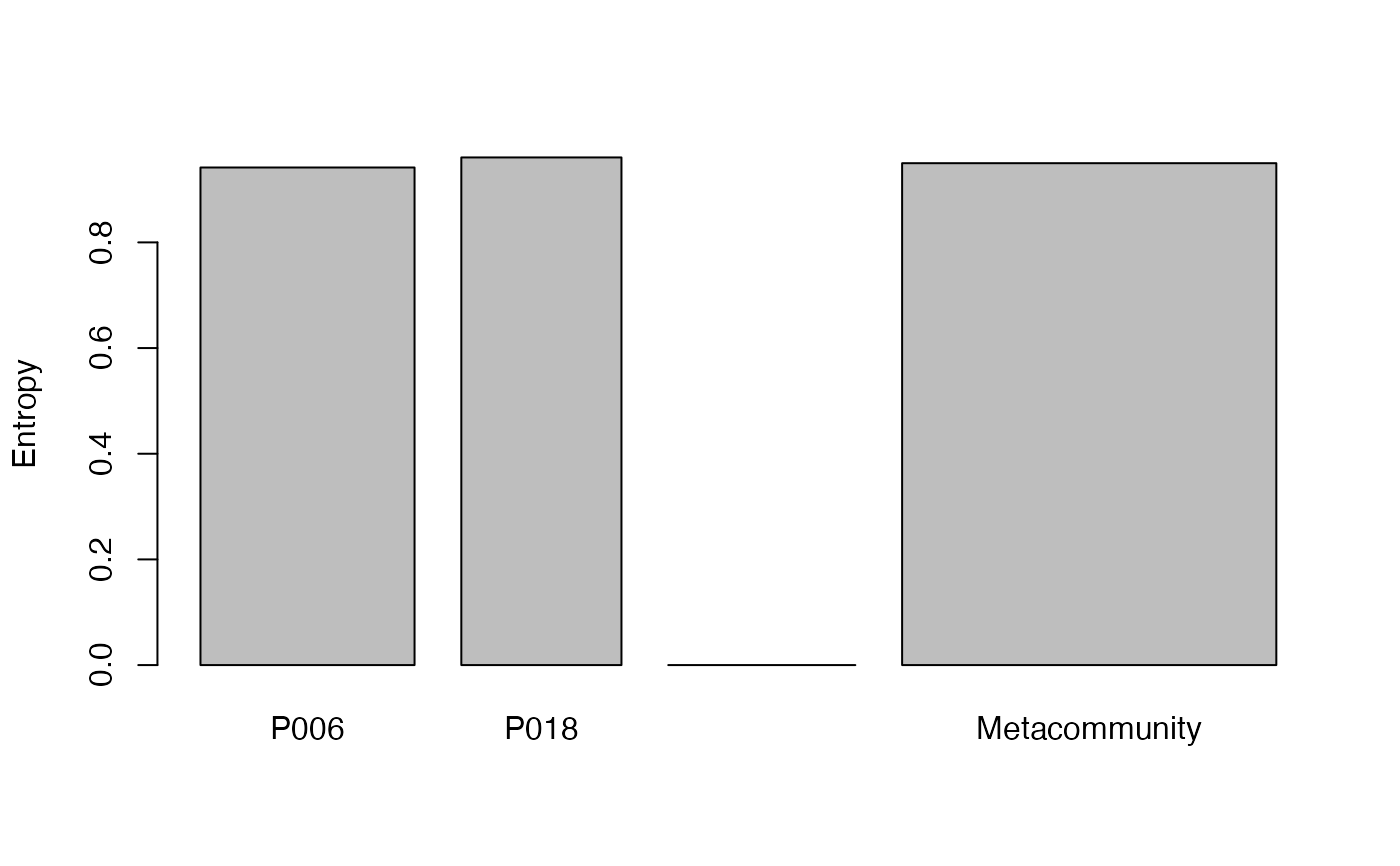Calculates the reduced-bias total alpha entropy of order $$q$$ of communities.

AlphaEntropy(MC, q = 1, Correction = "Best", Tree = NULL, Normalize = TRUE,
Z = NULL, CheckArguments = TRUE)

## Arguments

MC

A MetaCommunity object.

q

A number: the order of diversity. Default is 1 for Shannon entropy.

Correction

A string containing one of the possible corrections accepted by the bias-corrected entropy function (see details) or "None" or "Best", the default value.

Tree

An object of class hclust, phylo, phylog or PPtree. The tree must be ultrametric.

Normalize

If TRUE (default), the entropy returned by the function is normalized by the height of the tree (it is the weighted average value of the entropy in each slice).
If FALSE, it is the unnormalized weighted sum of the results.

Z

A relatedness matrix, i.e. a square matrix whose terms are all positive, strictly positive on the diagonal. Generally, the matrix is a similarity matrix, i.e. the diagonal terms equal 1 and other terms are between 0 and 1.

CheckArguments

Logical; if TRUE, the function arguments are verified. Should be set to FALSE to save time when the arguments have been checked elsewhere.

## Details

If Tree is not NULL, then phylogenetic entropy is calculated by bcPhyloEntropy; else, if Z is not NULL, then similarity-based entropy is calculated by bcHqz; else, neutral entropy is calculated by bcTsallis.

The alpha entropy of each community is calculated and summed according to community weights.

The possible corrections are detailed in Tsallis.

## Value

An MCentropy object containing entropy values of each community and of the metacommunity.

bcTsallis

## Examples

# Load Paracou data (number of trees per species in two 1-ha plot of a tropical forest)
data(Paracou618)
# Calculate Simpson alpha entropy
summary(AlphaEntropy(Paracou618.MC, 2))
#> Neutral alpha entropy of order 2 of metaCommunity Paracou618.MC
#> with correction: Best
#>
#> Entropy of communities:
#>      P006      P018
#> 0.9742146 0.9814741
#> Average entropy of the communities:
#>  0.9773212
# Compare without correction
summary(AlphaEntropy(Paracou618.MC, 2, Correction = "None"))
#> Neutral alpha entropy of order 2 of metaCommunity Paracou618.MC
#> with correction: None
#>
#> Entropy of communities:
#>      P006      P018
#> 0.9727197 0.9794563
#> Average entropy of the communities:
#>  0.9756026
# Estimate phylogenetic Simpson alpha entropy
summary(AlphaEntropy(Paracou618.MC, 2, Tree = Paracou618.Taxonomy) -> e)
#> HCDT alpha entropy of order 2 of metaCommunity Paracou618.MC with correction:
#> Best
#>
#> Phylogenetic or functional entropy was calculated according to the tree
#> Paracou618.Taxonomy
#>
#> Entropy is normalized
#> Entropy of communities:
#>      P006      P018
#> 0.9417393 0.9607787
#> Average entropy of the communities:
#>  0.9498869
plot(e)